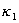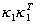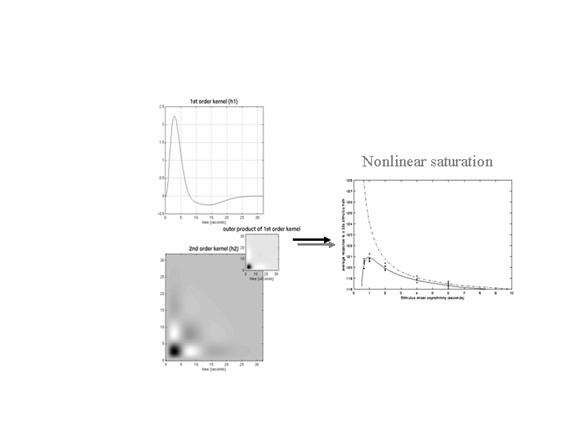Figure 11

Left panels: Volterra kernels from a voxel in the left superior temporal gyrus at -56, -28, 12mm. These kernel estimates were based on a single subject study of aural word presentation at different rates (from 0 to 90 words per minute) using a second order approximation to a Volterra series expansion modeling the observed hemodynamic response to stimulus input (a delta function for each word). These kernels can be thought of as a characterization of the second order hemodynamic response function. The first order kernel(upper panel) represents the (first order) component usually presented in linear analyses. The second order kernel (lower panel) is presented in image format. The color scale is arbitrary; white is positive and black is negative. The insert on the right represents, the second order kernel that would be predicted by a simple model that involved linear convolution withfollowed by some static nonlinearity.

Right panel: Integrated responses over a 32-second stimulus train as a function of SOA. Solid line: Estimates based on the nonlinear convolution model parameterized by the kernels on the left. Broken line: The responses expected in the absence of second order effects (i.e. in a truly linear system). Dots: Empirical averages based on the presentation of actual stimulus trains.